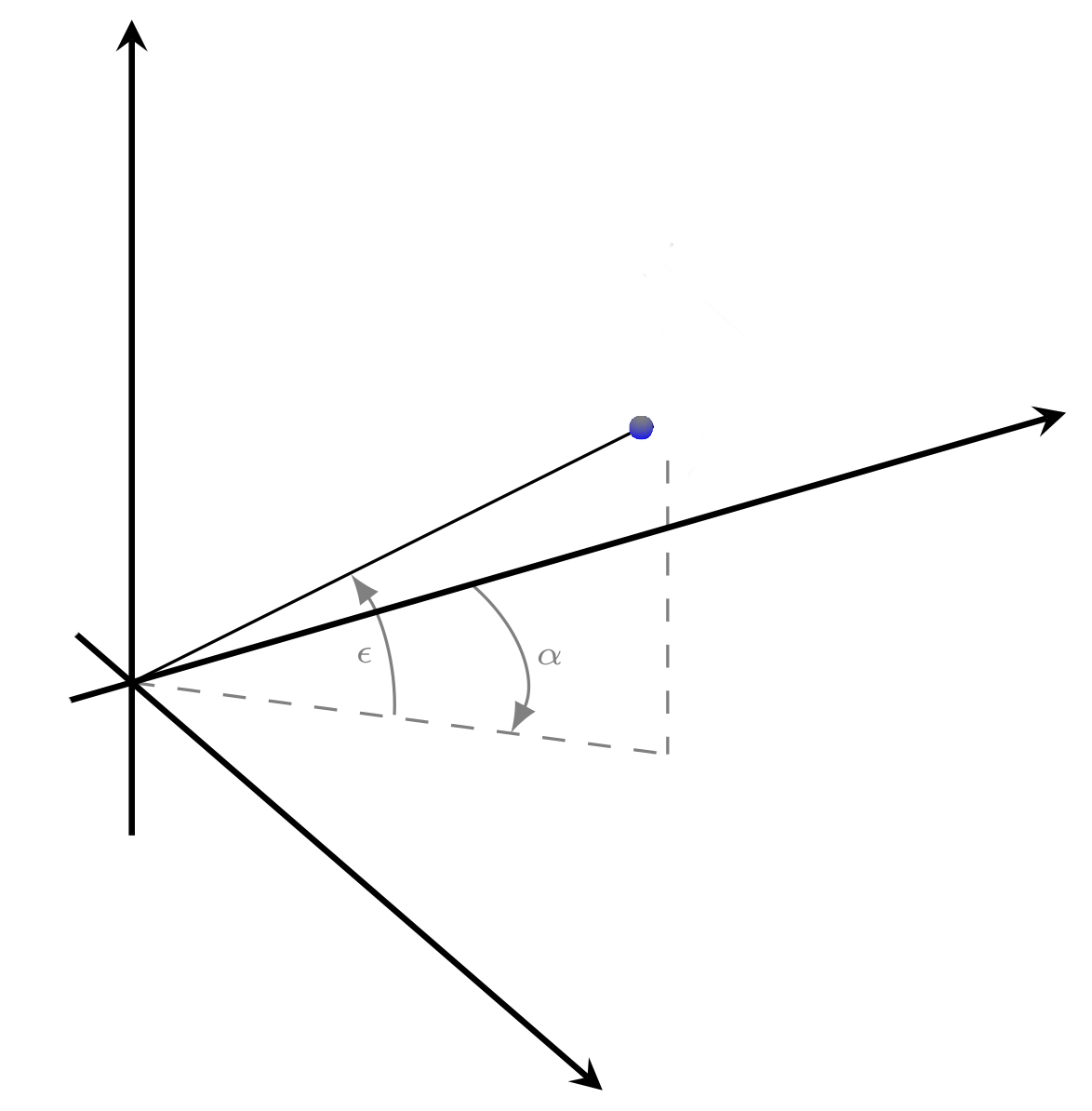# Encoding Ambisonics Sources

## The Virtual Source

The following example encodes a single monophonic audio signal to an Ambisonics source with two parameters:

• Azimuth: the horizontal angle of incidence.

• Elevation: the vertical angle of incidence.

Both angles are expressed in rad and range from $-\pi$ to $\pi$. Figure 1 shows a virtual sound source with these two parameters.Figure 1: Virtual sound source with two angles (azimuth and elevation).

## Encoding a 1st Order Source

### The Ambisonics Bus

First thing to create is an audio rate bus for the encoded Ambisonics signal. The bus size depends on the Ambisonics order M, following the formula $N = (M+1)^2$. For simplicity, this example uses first order:

s.boot;

// create the Ambisonics mix bus

~order     = 1;
~nHOA      = (pow(~order+1,2)).asInteger;
~ambi_BUS  = Bus.audio(s,~nHOA);


The channels of this bus correspond to the spherical harmonics. They encode the overall pressure and the distribution along the three basic dimensions. In the SC-HOA tools, Ambisonics channels are ordered according to the ACN convention and normalized with the N3D standard (Grond, 2017). The channels in the bus thus hold the three main axes in the following order:

Ambisonics channel ordering in the SC-HOA tools (ACN).

Spherical Harmonic Index

Channel

Description

1

W

omnidirectional

2

X

left-right

3

Z

top-bottom

4

Y

front-rear

### The Encoder

The SC-HOA library includes different encoders. This example uses the HOASphericalHarmonics class. This simple encoder can set the angles of incidence (azimuth, elevation) in spherical coordinates. Angles are controlled in radians:

• azimuth = 0 with elevation=0 is a signal straight ahead

• azimuth =-pi/2 is hard left

• azimuth = pi/2 is hard right

• azimuth = pi is in the back.

• elevation = pi/2 is on the top

• elevation = -pi/2 is on the bottom

This example uses a sawtooth signal as mono input and calculates the four Ambisonics channels.

~encoder_A = {arg azim=0, elev=0;
Out.ar(~ambi_BUS,HOASphericalHarmonics.coefN3D(~order,azim,elev)*Saw.ar(140));
}.play;


The Ambisonics bus can be monitored and the angles of the source can be set, manually:

~ambi_BUS.scope;

// set parameters
~encoder_A.set(\azim,0)
~encoder_A.set(\elev,0)


Exercise

Change the angles of the encoder and check whether the Ambisonics buses behave as expected. (Use multiples of pi/2.)

### The Decoder

The SC-HOA library features default binaural impulse responses, which need to be loaded first:

// load binaural IRs for the decoder


Afterwards, a first order HOABinaural decoder is fed with the encoded Ambisonics signal. It needs to be placed after the encoder node to get an audible output to the left and right channels. This output is the actual binaural signal for headphone use.

~decoder = {HOABinaural.ar(~order, In.ar(~ambi_BUS,~nHOA))}.play;
~decoder.moveAfter(~encoder_A);


Exercise

Listen to the decoded signal and change the angles.

## Panning Multiple Sources

Working with multiple sources requires a dedicated encoder for each source. All encoded signals are subsequently routed to the same Ambisonics bus and a single decoder is used to create the binaural signal. The angles of all sources can be set, individually.

~encoder_B = {arg azim=0, elev=0;
Out.ar(~ambi_BUS,HOASphericalHarmonics.coefN3D(~order,azim,elev)*Saw.ar(277))}.play;

~encoder_B.set(\azim,pi/4)
~encoder_B.set(\elev,1)


## Exercises

Exercise I

Use the mouse for a continuous control of a source's angles.

Exercise II

Add a control for the source distance to the encoder.

Exercise III

Increase the Ambisonics order and compare the results.

Exercise IV

Use OSC messages to control the positions of multiple sources.